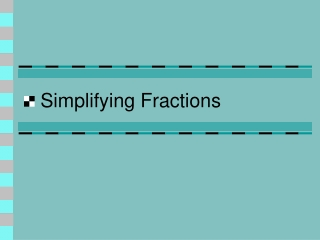DownloadDownload PresentationSimplifying Fractions

# Simplifying Fractions

Télécharger la présentation## Simplifying Fractions

- - - - - - - - - - - - - - - - - - - - - - - - - - - E N D - - - - - - - - - - - - - - - - - - - - - - - - - - -
##### Presentation Transcript

1. Simplifying Fractions

2. Vocabulary • Equivalent fractions – fractions that name the same number.

3. Equivalent Fractions

4. Equivalent Fractions • To find an equivalent fraction you multiply or divide the numerator and the denominator by the same number.

5. Equivalent Fractions x 2 = x 2

6. Equivalent Fractions  3 =  3

7. Vocabulary • Simplest form – when the GCF of the numerator and denominatoris one.

8. Rules for Simplifying Fractions • When the numerator is 1, the fraction will not reduce. • Example:

9. Rules for Simplifying Fractions • When the denominator is prime, the fraction will not reduce. • Example: (prime)

10. Rules for Simplifying Fractions • When the numerator is one less than the denominator, the fraction will not reduce. (Counting order) • Example:

11. Rules for Simplifying Fractions • When the numerator is prime and does not divide the denominator evenly, the fraction will not reduce. • Example: (prime)

12. Rules for Simplifying Fractions • When the numerator and the denominator are even, the fraction will always reduce. • Example:  2 =  2

13. Rules for Simplifying Fractions • When the numerator divides the denominator evenly, the fraction will always reduce. • Example:  5 =  5

14. Rules for Simplifying Fractions • When the numerator and the denominator can be divided by a common factor, the fraction will always reduce. • Example:  3 =  3